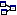» Tech Talk » Geek Stuff » binary math
 Geek Stuff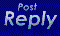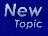binary math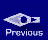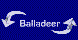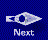Jeffrey Carter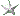Member Elite
since 2000-04-08
Posts 2367
State of constant confusion!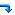0 posted 2004-07-29 07:01 PM

Anyone know any good websites on binary math?

Jeffrey CarterMember Elite
since 2000-04-08
Posts 2367
State of constant confusion!1 posted 2004-07-29 08:50 PM

ok i found this site http://www.cs.iupui.edu/~n241/readings/binconv.html but holy cow, anyone care to help me out .... this is hard stuff!!!
Ron
Member Rara Avis
since 1999-05-19
Posts 8669
Michigan, US2 posted 2004-07-29 10:31 PM

Binary math is easy. About ten times easier than decimal math, in fact.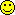When you add the digits 9 and 1, you know the answer can't be a digit greater than 9. So what do you do? You write down a zero and carry the one, and end up with 10.

What does this 10 mean? Since we're in base ten, it means no units and one ten.

When you add the digits 1 and 1 in a base two system, you know the answer can't be a digit greater than 1. So what do you do? You write down a zero and carry the one, and end up with 10.

What does this 10 mean? Since we're in base two, it means no units and one two.

When you see the number 1111 in the decimal system, you don't have to think real hard about what each digit really means, but that's only because it's been drilled into your head for so long. We know, without giving it much thought, that from right to left we have 1 unit of ones, 1 unit of tens, 1 unit of hundreds, and 1 unit of thousands. Each of those represents our base (ten) raised to a specific power.

1 * 10^0 = 1
1 * 10^1 = 10
1 * 10^2 = 100
1 * 10^3 = 1000

Add those all up an you get 1,111, or one thousand one hundred eleven. Simple stuff.

If you see that same 1111 in binary, you do EXACTLY the same thing.

1 * 2^0 = 1
1 * 2^1 = 2
1 * 2^2 = 4
1 * 2^3 = 8

Add those up and you'll know that binary 1111 is equivalent to decimal 15. It's *still* simple stuff.Jeffrey CarterMember Elite
since 2000-04-08
Posts 2367
State of constant confusion!3 posted 2004-07-30 01:49 PM

so instead of raising the number to a power of 10 we raise it to a power of 2?

so in binary the number 1101 would be written like this....

1*2^0=1
1*2^1=2 but actually since the second number is zero the end result would be zero also correct?
1*2^2=4
1*2^3=8

so the end result would be 13?

Thanks for taking the time to help me with this Ron, I really appreciate it.

Ron
Member Rara Avis
since 1999-05-19
Posts 8669
Michigan, US4 posted 2004-07-30 07:50 PM

You've got the right idea (and the right answer), Jeffrey, but still need just one minor adjustment. Change your example to this:

1*2^0=1
0*2^1=0
1*2^2=4
1*2^3=8

Notice how the digits on the left now reflect the 1101 you are calculating? Another example in decimal might make it more clear why:

0*10^0 = 0*1 = 0
2*10^1 = 2*10 = 20
7*10^2 = 7*100 = 700
5*10^3 = 5*1000 = 5000

Ergo, we know 5720 in base 10 is, uh, 5,720.(For those who might be wondering, any number raised to a power of zero equals one, and any number raised to a power of one will equal itself.)

Jeffrey CarterMember Elite
since 2000-04-08
Posts 2367
State of constant confusion!5 posted 2004-07-31 12:06 PM

ok, Ron, thanks again for taking the time to help me I really do appreciate it.All times are ET (US). All dates are in Year-Month-Day format.» Tech Talk » Geek Stuff » binary math